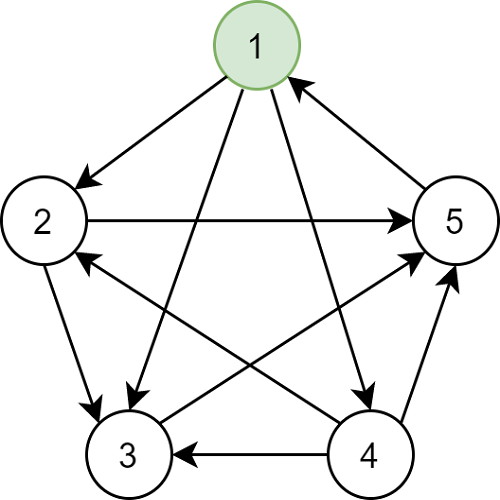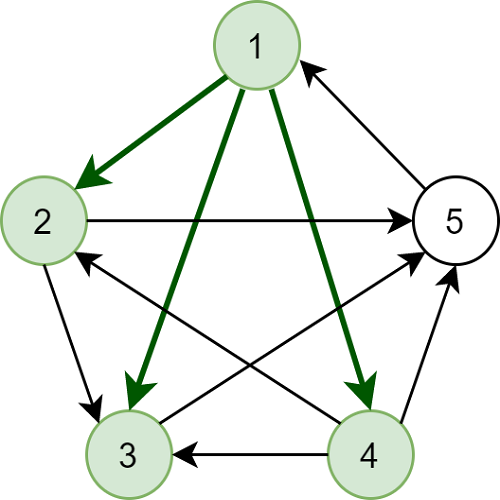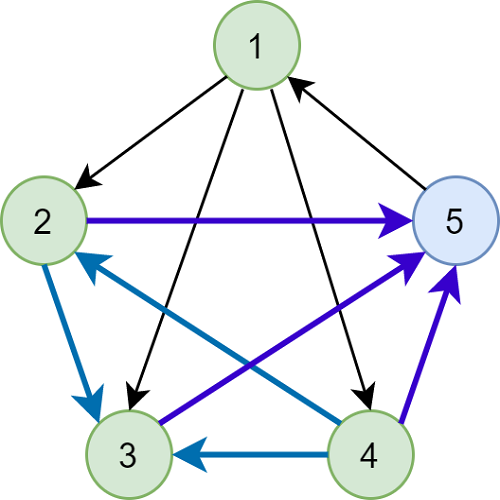# Drexel University

College of Computing and Informatics

## A King Independent Set in every Pair of Transformed Graphs

`Research`
`Graph theoretical problem with applications in distributed computing and networking``We are given a connected undirected graph G(V, E) and two transformations A and B that both apply a direction to each edge in E. We describe a graph covering algorithm for this problem in the following way: starting with an independent set S of vertices from V, we cover every adjacent vertex to the vertices in S, in the directed graph resulted from applying transformation A to E, i.e. G_1 = (V, A(E)); we then cover every adjacent vertex to all vertices already covered (including S), in the directed graph resulted from applying transformation B to E, i.e. G_2 = (V, B(E)). The goal is to prove that for any graph G and any transformations A and B, there always exists an independent set of vertices S such that this algorithm covers every vertex at least once. This paper studies multiple graph structures, such as complete, bipartite, k-partite, as well as some algorithms and techniques used to make observations about the properties of these graphs as they can be applied to the graph covering problem.`### Team Members### Behind The Scenes